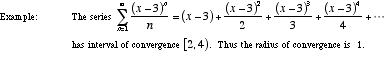index: click on a letter A B C D E F G H I J K L M N O P Q R S T U V W X Y Z A to Z index index: subject areas numbers & symbols sets, logic, proofs geometry algebra trigonometry advanced algebra & pre-calculus calculus advanced topics probability & statistics real world applications multimedia entrieswww.mathwords.com about mathwords website feedback

 Radius of Convergence The distance between the center of a power series' interval of convergence and its endpoints. If the series only converges at a single point, the radius of convergence is 0. If the series converges over all real numbers, the radius of convergence is ∞.See also